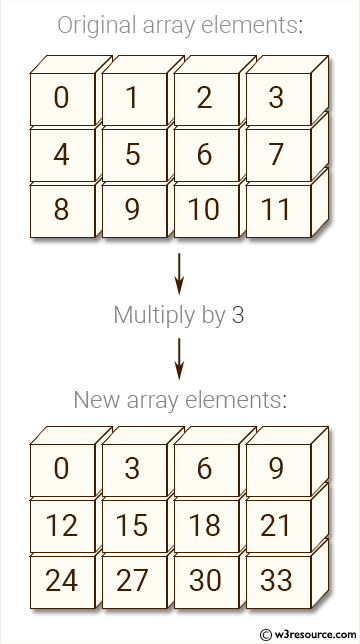﻿ NumPy: Create an array of (3, 4) shape, multiply every element value by 3 and display the new array - w3resource# NumPy: Create an array of (3, 4) shape, multiply every element value by 3 and display the new array

## NumPy: Array Object Exercise-73 with Solution

Write a NumPy program to create an array of (3, 4) shape, multiply every element value by 3 and display the new array.

Pictorial Presentation:Sample Solution:

Python Code:

``````import numpy as np
x= np.arange(12).reshape(3, 4)
print("Original array elements:")
print(x)
a[...] = 3 * a
print("New array elements:")
print(x)
```
```

Sample Output:

```Original array elements:
[[ 0  1  2  3]
[ 4  5  6  7]
[ 8  9 10 11]]
New array elements:
[[ 0  3  6  9]
[12 15 18 21]
[24 27 30 33]]
```

Python Code Editor:

Have another way to solve this solution? Contribute your code (and comments) through Disqus.

What is the difficulty level of this exercise?

Test your Python skills with w3resource's quiz

﻿

## Python: Tips of the Day

List comprehension:

```>>> m = [x ** 2 for x in range(5)]
>>> m
[0, 1, 4, 9, 16]
```